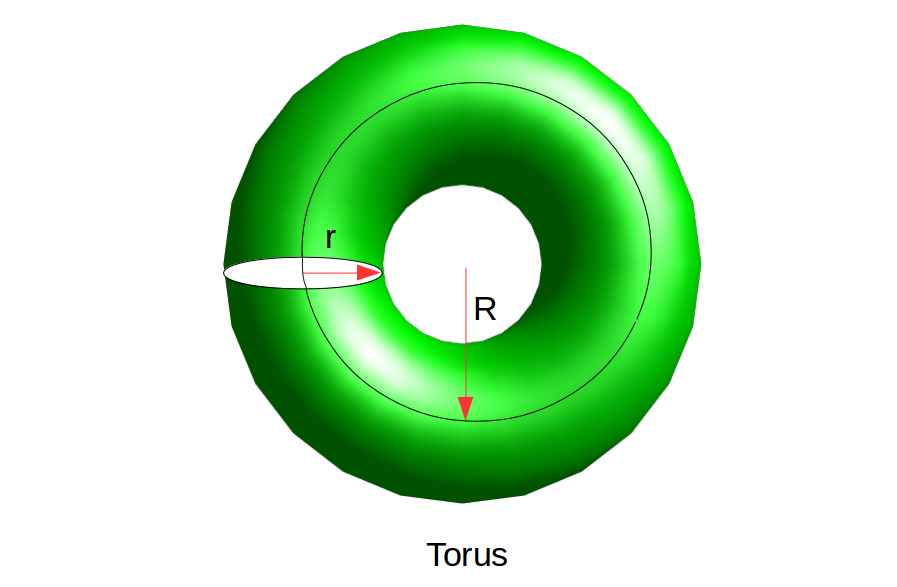GeeksforGeeks App
Open AppBrowser
Continue

# Calculate volume and surface area of TorusTorus

Property:

2. It is not a polyhedron
3. It has no vertices or edges
• Surface Area
The surface area of a Torus is given by the formula –
`Surface Area = 4 × Pi^2 × R × r`
• Where r is the radius of the small circle and R is the radius of bigger circle and Pi is constant Pi=3.14159.

• Volume
The volume of a cone is given by the formula –
`Volume = 2 × Pi^2 × R × r^2`
• Where r is the radius of the small circle and R is the radius of bigger circle and Pi is constant Pi=3.14159.

Examples:

```Input : r=3, R=7
Output :
Volume: 1243.568195
Surface: 829.045464```

## C++

 `// C++ program to calculate volume``// and surface area of Torus``#include``using` `namespace` `std;` `int` `main()``{``    ``// radius of inner circle``    ``double` `r = 3;` `    ``// distance from origin to center of inner circle``    ``// radius of black circle in figure``    ``double` `R = 7;` `    ``// Value of Pi``    ``float` `pi = (``float``)3.14159;``    ``double` `Volume = 0;``    ``Volume = 2 * pi * pi * R * r * r;``    ``cout<<``"Volume: "``<

## C

 `// C program to calculate volume``// and surface area of Torus``#include ``int` `main()``{``    ``// radius of inner circle``    ``double` `r = 3;` `    ``// distance from origin to center of inner circle``    ``// radius of black circle in figure``    ``double` `R = 7;` `    ``// Value of Pi``    ``float` `pi = (``float``)3.14159;``    ``double` `Volume = 0;``    ``Volume = 2 * pi * pi * R * r * r;``    ``printf``(``"Volume: %f"``, Volume);` `    ``double` `Surface = 4 * pi * pi * R * r;``    ``printf``(``"\nSurface: %f"``, Surface);``}`

## Java

 `// Java program to calculate volume``// and surface area of Torus``class` `Test {` `    ``public` `static` `void` `main(String args[])``    ``{` `        ``// radius of inner circle``        ``double` `r = ``3``;` `        ``// distance from origin to center of inner circle``        ``// radius of black circle in figure``        ``double` `R = ``7``;` `        ``// Value of Pi``        ``float` `pi = (``float``)``3.14159``;``        ``double` `Volume = ``0``;``        ``Volume = ``2` `* pi * pi * R * r * r;``        ``System.out.printf(``"Volume: %f"``, Volume);` `        ``double` `Surface = ``4` `* pi * pi * R * r;``        ``System.out.printf(``"\nSurface: %f"``, Surface);``    ``}``}`

## Python3

 `# Python3 program to calculate volume``# and surface area of Torus``# radius of inner circle``r ``=` `3` `# distance from origin to center of inner circle``# radius of black circle in figure``R ``=` `7` `# Value of Pi``pi ``=` `3.14159``Volume ``=` `(``float``)(``2` `*` `pi ``*` `pi ``*` `R ``*` `r ``*` `r);``print``(``"Volume: "``, Volume);``Surface ``=` `(``float``)(``4` `*` `pi ``*` `pi ``*` `R ``*` `r);``print``(``"Surface: "``, Surface);`

## C#

 `// C# program to calculate volume``// and surface area of Torus``using` `System;` `class` `GFG``{``    ` `// Driver Code``public` `static` `void` `Main()``{` `    ``// radius of inner circle``    ``double` `r = 3;` `    ``// distance from origin to center``    ``// of inner circle radius of black``    ``// circle in figure``    ``double` `R = 7;` `    ``// Value of Pi``    ``float` `pi = (``float``)3.14159;``    ``double` `Volume = 0;``    ``Volume = 2 * pi * pi * R * r * r;``    ``Console.WriteLine(``"Volume: {0}"``, Volume);` `    ``double` `Surface = 4 * pi * pi * R * r;``    ``Console.WriteLine(``"Surface: {0}"``, Surface);``}``}` `// This code is contributed by Soumik`

## PHP

 ``

## Javascript

 ``

Output:

```Volume: 1243.568195
Surface: 829.045464```

Time complexity : O(1)
Auxiliary Space : O(1)

My Personal Notes arrow_drop_up